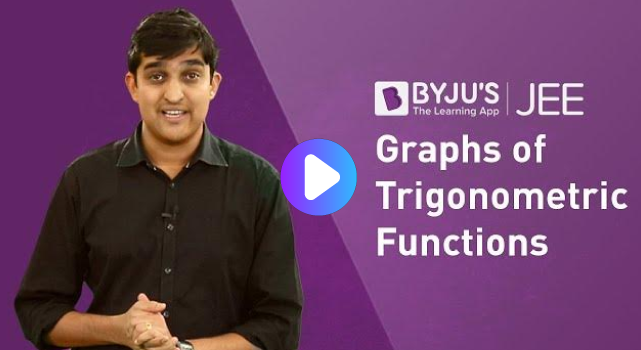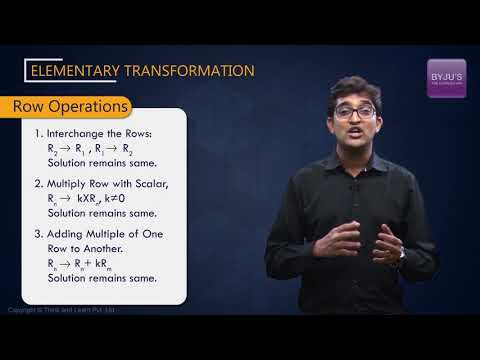# ICSE Class 10 Maths

Mathematics is a challenging subject and is a nightmare for many class 10 students. The vast syllabus, formulae, and concepts add up to the fear as well. The fun fact is that math isn’t complicated. All that is required to score good marks in Maths is a thorough understanding of concepts and practice. Students should have a good knowledge of the syllabus and the concepts to be learned, to overcome the fear of mathematics. This will help the students in determining the time required to practise them, considering their difficulty level.

## ICSE Class 10 Maths Chapters

 Value Added Tax Similarity Banking Loci Shares and Dividend Circle: Arc and Cyclic Properties Linear Inequations Circle: Tangents and Intersecting Chords Quadratic Equations Circle: Constructions Ratio and Proportion Mensuration: Cylinder, Cone and Sphere Remainder and Factor Theorems Trigonometrical Identities Matrices Height and Distance Arithmetic Progression Statistics: Graphical Representation Geometric Progression Measures of Central Tendency – Mean Coordinate Geometry – Reflection Measures of Central Tendency – Median and Mode Section and Mid-Point Formula Probability Equation of a Line

## ICSE Class 10 Maths Syllabus

The CISCE Board prescribes the syllabus of ICSE Class 10 Maths. The syllabus helps students to know about the topics and concepts they are going to study for that respective academic year. Along with the course content, it contains information about time duration, distribution of marks, etc. Students should go through the entire syllabus before starting their exam preparation.

## ICSE Class 10 Maths Previous Year Paper

Students should solve ICSE Class 10 Maths Previous Year Paper to know the exact question paper pattern, weightage of marks, important topics and time duration. These previous year question paper are prepared according to the ICSE Class 10 Maths syllabus. Solve question paper of different years to improve your overall performance and work on your weak points.

## ICSE Class 10 Maths Selina Solutions

Our subject experts prepare ICSE Class 10 Maths Selina Solutions in accordance with the Class 10 Maths syllabus. Students can refer to these solutions whenever they have any doubt while solving the Concise Selina textbook questions. The solutions are explained in a proper stepwise format using simple language so that students can understand it easily. It is considered as the best reference material while revising the entire syllabus.

## ICSE Class 10 ML Aggarwal Solutions

ICSE Class 10 Maths ML Aggarwal Solutions are created by expert faculty at BYJU’S as per the Class 10 ICSE Maths Syllabus. Students can easily tackle tricky problems using the solutions PDF provided as a major reference material. The questions are formulated in an understandable way, helping the students solve problems effortlessly. These solutions PDF help students of Class 10 in obtaining a strong foundation, so as to score well in the final exam.

## ICSE Class 10 Maths Sample Paper

ICSE Class 10 Maths Sample Paper covers the entire syllabus and provides different types of questions for students to practice. It also gives an idea about the important concepts, formulas, weightage of marks, etc. Practising questions from sample papers will help students to gain their confidence which in turn will help to fetch them good marks. Practice it on a regular basis once you are done with the entire syllabus.

## ICSE Class 10 Maths Important Questions

The important questions of ICSE Class 10 Maths help students to practice important numerical problems from each chapter. By doing so, they can cover the entire syllabus in quick time before the exam. These important questions also provide the students with a brief knowledge of the question pattern, essential topics, and important question type. By following ICSE Class 10 Maths important questions, students can evaluate their performance and preparations so far.

## ICSE Class 10 Maths Videos

ICSE Class 10 Maths videos explain all the concepts in a very simple way so that students can understand in a better way. Learning through video will help students to clear their concepts easily as it is explained with suitable and illustrative examples. Our subject experts explain difficult concepts by referring example of our daily activities.### How to prepare for ICSE Class 10 Maths Examination?

1. Dedicate at least 3 hours a day to study the topics taught that day.
2. Solve as many sample papers possible to get an idea of the questions that could be asked.
3. Practice numerical problems.
4. Solve previous year question papers and get an idea of the repeated questions.